# Cosine Function

The cosine function is a periodic function which is very important in trigonometry.

The simplest way to understand the cosine function is to use the unit circle. For a given angle measure $\theta$ , draw a unit circle on the coordinate plane and draw the angle centered at the origin, with one side as the positive  $x$ -axis. The $x$ -coordinate of the point where the other side of the angle intersects the circle is $\mathrm{cos}\left(\theta \right)$ , and the  $y$ -coordinate is $\mathrm{sin}\left(\theta \right)$ .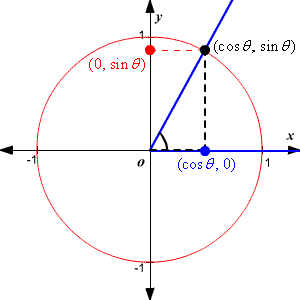There are a few cosine values that should be memorized, based on  $30°-60°-90°$ triangles  and  $45°-45°-90°$ triangles .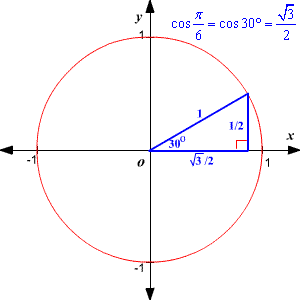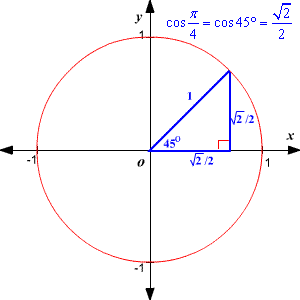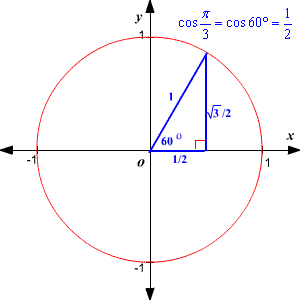Once you know these values, you can derive many other values for the cosine function. Remember that cos\theta; is positive in quadrants $\text{I}$ and $\text{I}\text{V}$ and negative in quadrants $\text{I}\text{I}$ and $\text{I}\text{I}\text{I}$ .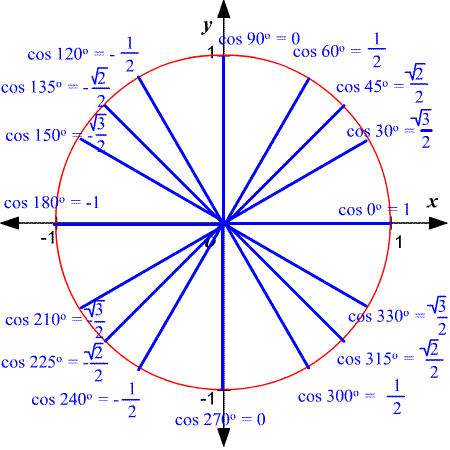You can plot these points on a coordinate plane to show part of the cosine function, the part between $0$ and $2\pi$ .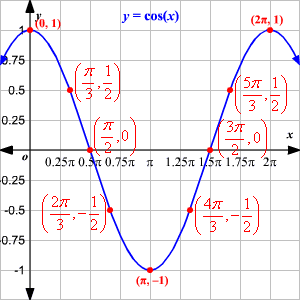For values of $\theta$ less than $0$ or greater than $2\pi$ you can find the value of $\mathrm{cos}\left(\theta \right)$ using the reference angle .

The graph of the function over a wider interval is shown below.Note that the of the function is the whole real line, while the range is $-1\le y\le 1$ .

The period of $f\left(x\right)=\mathrm{cos}\left(x\right)$ is $2\pi$ . That is, the shape of the curve repeats every $2\pi$ -unit interval on the $x$ -axis.

The amplitude of $f\left(x\right)=\mathrm{cos}\left(x\right)$ is $1$ , that is, the height of the wave.

The modified function $y=a\mathrm{cos}\left(bx\right)$ has amplitude $a$ and period $2\pi$ / $b$ .